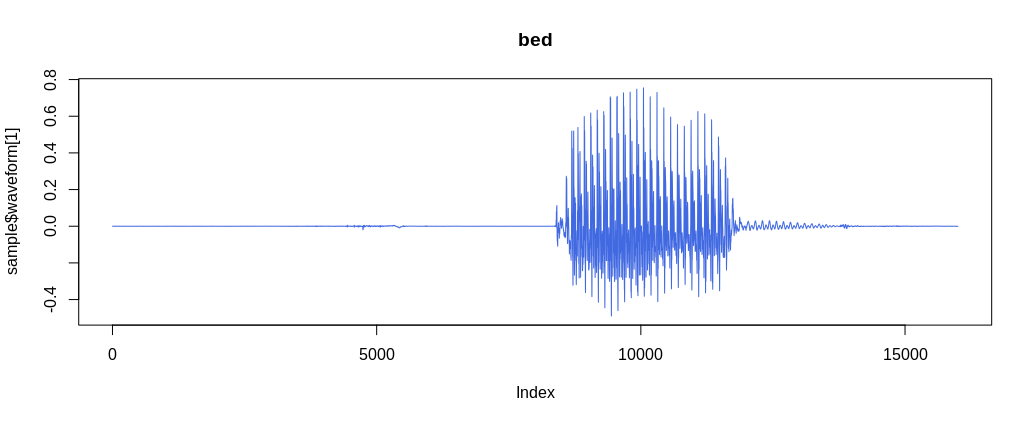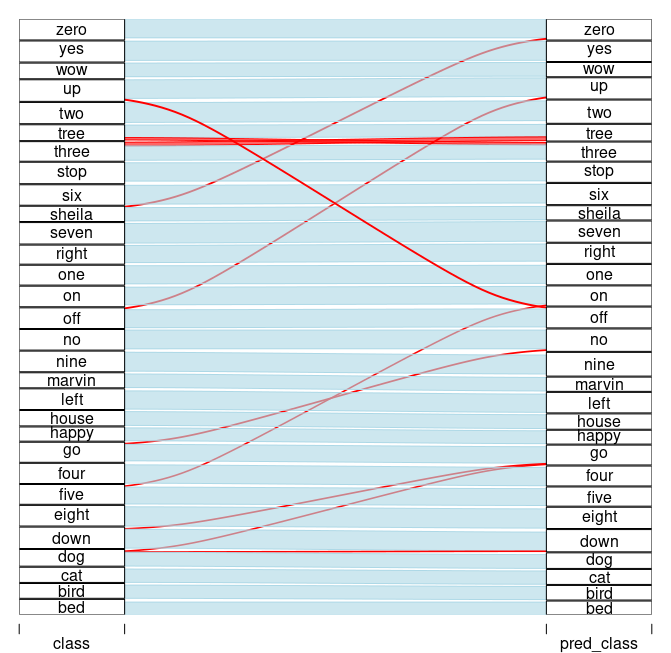Categories

# Simple audio classification with torch

This article translates Daniel Falbel’s ‘Simple Audio Classification’ article from tensorflow/keras to torch/torchaudio. The main goal is to introduce torchaudio and illustrate its contributions to the torch ecosystem. Here, we focus on a popular dataset, the audio loader and the spectrogram transformer. An interesting side product is the parallel between torch and tensorflow, showing sometimes the differences, sometimes the similarities between them.

```library(torch)
library(torchaudio)
```

torchaudio has the speechcommand_dataset built in. It filters out background_noise by default and lets us choose between versions v0.01 and v0.02.

```# set an existing folder here to cache the dataset
DATASETS_PATH <- "~/datasets/"

df <- speechcommand_dataset(
root = DATASETS_PATH,
url = "speech_commands_v0.01",
)

# expect folder: _background_noise_
df\$EXCEPT_FOLDER
#  "_background_noise_"

# number of audio files
length(df)
#  64721

# a sample
sample <- df

sample\$waveform[, 1:10]
```
```torch_tensor
0.0001 *
0.9155  0.3052  1.8311  1.8311 -0.3052  0.3052  2.4414  0.9155 -0.9155 -0.6104
[ CPUFloatType{1,10} ]
```
```sample\$sample_rate
# 16000
sample\$label
# bed

plot(sample\$waveform, type = "l", col = "royalblue", main = sample\$label)
```(#fig:unnamed-chunk-4)A sample waveform for a ‘bed’.

Classes

```df\$classes
```
```  "bed"    "bird"   "cat"    "dog"    "down"   "eight"  "five"
 "four"   "go"     "happy"  "house"  "left"   "marvin" "nine"
 "no"     "off"    "on"     "one"    "right"  "seven"  "sheila"
 "six"    "stop"   "three"  "tree"   "two"    "up"     "wow"
 "yes"    "zero"
```

torch::dataloader has the same task as data_generator defined in the original article. It is responsible for preparing batches – including shuffling, padding, one-hot encoding, etc. – and for taking care of parallelism / device I/O orchestration.

In torch we do this by passing the train/test subset to torch::dataloader and encapsulating all the batch setup logic inside a collate_fn() function.

```set.seed(6)
id_train <- sample(length(df), size = 0.7*length(df))
id_test <- setdiff(seq_len(length(df)), id_train)
# subsets

train_subset <- torch::dataset_subset(df, id_train)
test_subset <- torch::dataset_subset(df, id_test)
```

At this point, dataloader(train_subset) would not work because the samples are not padded. So we need to build our own collate_fn() with the padding strategy.

I suggest using the following approach when implementing the collate_fn():

1. begin with collate_fn <- function(batch) browser().
2. instantiate dataloader with the collate_fn()
3. create an environment by calling enumerate(dataloader) so you can ask to retrieve a batch from dataloader.
4. run environment[][]. Now you should be sent inside collate_fn() with access to batch input object.
5. build the logic.
```collate_fn <- function(batch) {
browser()
}

train_subset,
batch_size = 32,
shuffle = TRUE,
collate_fn = collate_fn
)

ds_train_env <- enumerate(ds_train)
ds_train_env[][]
```

The final collate_fn() pads the waveform to length 16001 and then stacks everything up together. At this point there are no spectrograms yet. We going to make spectrogram transformation a part of model architecture.

```pad_sequence <- function(batch) {
# Make all tensors in a batch the same length by padding with zeros
batch <- sapply(batch, function(x) (x\$t()))
return(batch\$permute(c(1, 3, 2)))
}

# Final collate_fn
collate_fn <- function(batch) {
# Input structure:
# list of 32 lists: list(waveform, sample_rate, label, speaker_id, utterance_number)
# Transpose it
batch <- purrr::transpose(batch)
tensors <- batch\$waveform
targets <- batch\$label_index

# Group the list of tensors into a batched tensor

# target encoding
targets <- torch::torch_stack(targets)

list(tensors = tensors, targets = targets) # (64, 1, 16001)
}
```

Batch structure is:

• batch[]: waveforms – tensor with dimension (32, 1, 16001)
• batch[]: targets – tensor with dimension (32, 1)

Also, torchaudio comes with 3 loaders, av_loader, tuner_loader, and audiofile_loader- more to come. set_audio_backend() is used to set one of them as the audio loader. Their performances differ based on audio format (mp3 or wav). There is no perfect world yet: tuner_loader is best for mp3, audiofile_loader is best for wav, but neither of them has the option of partially loading a sample from an audio file without bringing all the data into memory first.

For a given audio backend we need pass it to each worker through worker_init_fn() argument.

```ds_train <- dataloader(
train_subset,
batch_size = 128,
shuffle = TRUE,
collate_fn = collate_fn,
num_workers = 16,
)

test_subset,
batch_size = 64,
shuffle = FALSE,
collate_fn = collate_fn,
num_workers = 8,
)
```

Model definition

Instead of keras::keras_model_sequential(), we are going to define a torch::nn_module(). As referenced by the original article, the model is based on this architecture for MNIST from this tutorial, and I’ll call it ‘DanielNN’.

```dan_nn <- torch::nn_module(
"DanielNN",

initialize = function(
window_size_ms = 30,
window_stride_ms = 10
) {

# spectrogram spec
window_size <- as.integer(16000*window_size_ms/1000)
stride <- as.integer(16000*window_stride_ms/1000)
fft_size <- as.integer(2^trunc(log(window_size, 2) + 1))
n_chunks <- length(seq(0, 16000, stride))

self\$spectrogram <- torchaudio::transform_spectrogram(
n_fft = fft_size,
win_length = window_size,
hop_length = stride,
normalized = TRUE,
power = 2
)

# convs 2D
self\$conv1 <- torch::nn_conv2d(in_channels = 1, out_channels = 32, kernel_size = c(3,3))
self\$conv2 <- torch::nn_conv2d(in_channels = 32, out_channels = 64, kernel_size = c(3,3))
self\$conv3 <- torch::nn_conv2d(in_channels = 64, out_channels = 128, kernel_size = c(3,3))
self\$conv4 <- torch::nn_conv2d(in_channels = 128, out_channels = 256, kernel_size = c(3,3))

# denses
self\$dense1 <- torch::nn_linear(in_features = 14336, out_features = 128)
self\$dense2 <- torch::nn_linear(in_features = 128, out_features = 30)
},

forward = function(x) {
x %>% # (64, 1, 16001)
self\$spectrogram() %>% # (64, 1, 257, 101)
torch::torch_log() %>%
self\$conv1() %>%
torch::nnf_relu() %>%
torch::nnf_max_pool2d(kernel_size = c(2,2)) %>%

self\$conv2() %>%
torch::nnf_relu() %>%
torch::nnf_max_pool2d(kernel_size = c(2,2)) %>%

self\$conv3() %>%
torch::nnf_relu() %>%
torch::nnf_max_pool2d(kernel_size = c(2,2)) %>%

self\$conv4() %>%
torch::nnf_relu() %>%
torch::nnf_max_pool2d(kernel_size = c(2,2)) %>%

torch::nnf_dropout(p = 0.25) %>%
torch::torch_flatten(start_dim = 2) %>%

self\$dense1() %>%
torch::nnf_relu() %>%
torch::nnf_dropout(p = 0.5) %>%
self\$dense2()
}
)

model <- dan_nn()

device <- torch::torch_device(if(torch::cuda_is_available()) "cuda" else "cpu")
model\$to(device = device)

print(model)
```
```An `nn_module` containing 2,226,846 parameters.

── Modules ──────────────────────────────────────────────────────
● spectrogram: <Spectrogram> #0 parameters
● conv1: <nn_conv2d> #320 parameters
● conv2: <nn_conv2d> #18,496 parameters
● conv3: <nn_conv2d> #73,856 parameters
● conv4: <nn_conv2d> #295,168 parameters
● dense1: <nn_linear> #1,835,136 parameters
● dense2: <nn_linear> #3,870 parameters
```

Model fitting

Unlike in tensorflow, there is no model %>% compile(…) step in torch, so we are going to set loss criterion, optimizer strategy and evaluation metrics explicitly in the training loop.

```loss_criterion <- torch::nn_cross_entropy_loss()
metrics <- list(acc = yardstick::accuracy_vec)
```

Training loop

```library(glue)
library(progress)

pred_to_r <- function(x) {
classes <- factor(df\$classes)
classes[as.numeric(x\$to(device = "cpu"))]
}

set_progress_bar <- function(total) {
progress_bar\$new(
total = total, clear = FALSE, width = 70,
format = ":current/:total [:bar] - :elapsed - loss: :loss - acc: :acc"
)
}
```
```epochs <- 20
losses <- c()
accs <- c()

for(epoch in seq_len(epochs)) {
pb <- set_progress_bar(length(ds_train))
pb\$message(glue("Epoch {epoch}/{epochs}"))
coro::loop(for(batch in ds_train) {
predictions <- model(batch[]\$to(device = device))
targets <- batch[]\$to(device = device)
loss <- loss_criterion(predictions, targets)
loss\$backward()
optimizer\$step()

# eval reports
prediction_r <- pred_to_r(predictions\$argmax(dim = 2))
targets_r <- pred_to_r(targets)
acc <- metrics\$acc(targets_r, prediction_r)
accs <- c(accs, acc)
loss_r <- as.numeric(loss\$item())
losses <- c(losses, loss_r)

pb\$tick(tokens = list(loss = round(mean(losses), 4), acc = round(mean(accs), 4)))
})
}

# test
predictions_r <- c()
targets_r <- c()
coro::loop(for(batch_test in ds_test) {
predictions <- model(batch_test[]\$to(device = device))
targets <- batch_test[]\$to(device = device)
predictions_r <- c(predictions_r, pred_to_r(predictions\$argmax(dim = 2)))
targets_r <- c(targets_r, pred_to_r(targets))
})
val_acc <- metrics\$acc(factor(targets_r, levels = 1:30), factor(predictions_r, levels = 1:30))
cat(glue("val_acc: {val_acc}nn"))
```
```Epoch 1/20
[W SpectralOps.cpp:590] Warning: The function torch.rfft is deprecated and will be removed in a future PyTorch release. Use the new torch.fft module functions, instead, by importing torch.fft and calling torch.fft.fft or torch.fft.rfft. (function operator())
354/354 [=========================] -  1m - loss: 2.6102 - acc: 0.2333
Epoch 2/20
354/354 [=========================] -  1m - loss: 1.9779 - acc: 0.4138
Epoch 3/20
354/354 [============================] -  1m - loss: 1.62 - acc: 0.519
Epoch 4/20
354/354 [=========================] -  1m - loss: 1.3926 - acc: 0.5859
Epoch 5/20
354/354 [==========================] -  1m - loss: 1.2334 - acc: 0.633
Epoch 6/20
354/354 [=========================] -  1m - loss: 1.1135 - acc: 0.6685
Epoch 7/20
354/354 [=========================] -  1m - loss: 1.0199 - acc: 0.6961
Epoch 8/20
354/354 [=========================] -  1m - loss: 0.9444 - acc: 0.7181
Epoch 9/20
354/354 [=========================] -  1m - loss: 0.8816 - acc: 0.7365
Epoch 10/20
354/354 [=========================] -  1m - loss: 0.8278 - acc: 0.7524
Epoch 11/20
354/354 [=========================] -  1m - loss: 0.7818 - acc: 0.7659
Epoch 12/20
354/354 [=========================] -  1m - loss: 0.7413 - acc: 0.7778
Epoch 13/20
354/354 [=========================] -  1m - loss: 0.7064 - acc: 0.7881
Epoch 14/20
354/354 [=========================] -  1m - loss: 0.6751 - acc: 0.7974
Epoch 15/20
354/354 [=========================] -  1m - loss: 0.6469 - acc: 0.8058
Epoch 16/20
354/354 [=========================] -  1m - loss: 0.6216 - acc: 0.8133
Epoch 17/20
354/354 [=========================] -  1m - loss: 0.5985 - acc: 0.8202
Epoch 18/20
354/354 [=========================] -  1m - loss: 0.5774 - acc: 0.8263
Epoch 19/20
354/354 [==========================] -  1m - loss: 0.5582 - acc: 0.832
Epoch 20/20
354/354 [=========================] -  1m - loss: 0.5403 - acc: 0.8374
val_acc: 0.876705979296493
```

Making predictions

We already have all predictions calculated for test_subset, let’s recreate the alluvial plot from the original article.

```library(dplyr)
library(alluvial)
df_validation <- data.frame(
pred_class = df\$classes[predictions_r],
class = df\$classes[targets_r]
)
x <-  df_validation %>%
mutate(correct = pred_class == class) %>%
count(pred_class, class, correct)

alluvial(
x %>% select(class, pred_class),
freq = x\$n,
col = ifelse(x\$correct, "lightblue", "red"),
border = ifelse(x\$correct, "lightblue", "red"),
alpha = 0.6,
hide = x\$n < 20
)
```(#fig:unnamed-chunk-15)Model performance: true labels <–> predicted labels.

Model accuracy is 87,7%, somewhat worse than tensorflow version from the original post. Nevertheless, all conclusions from original post still hold.